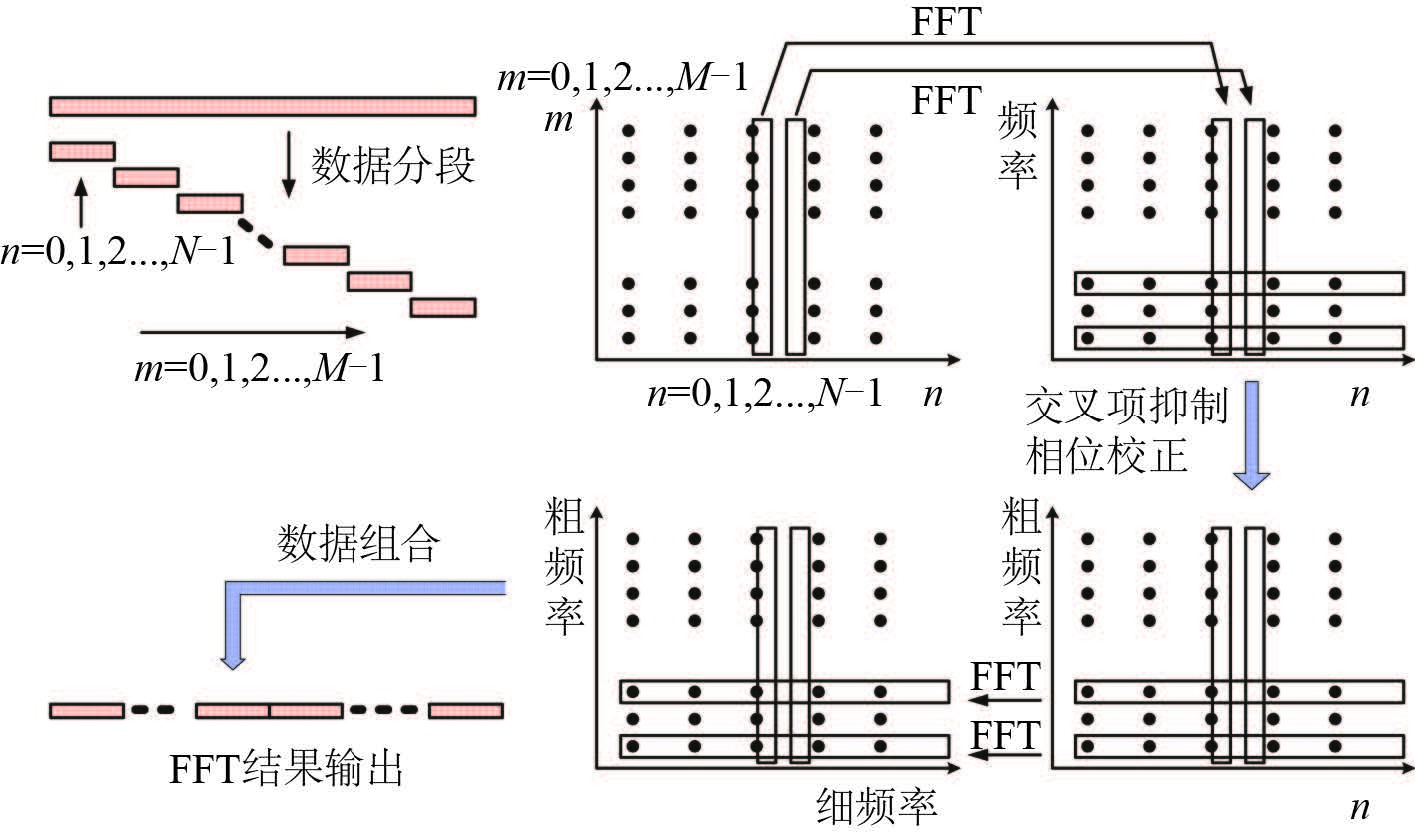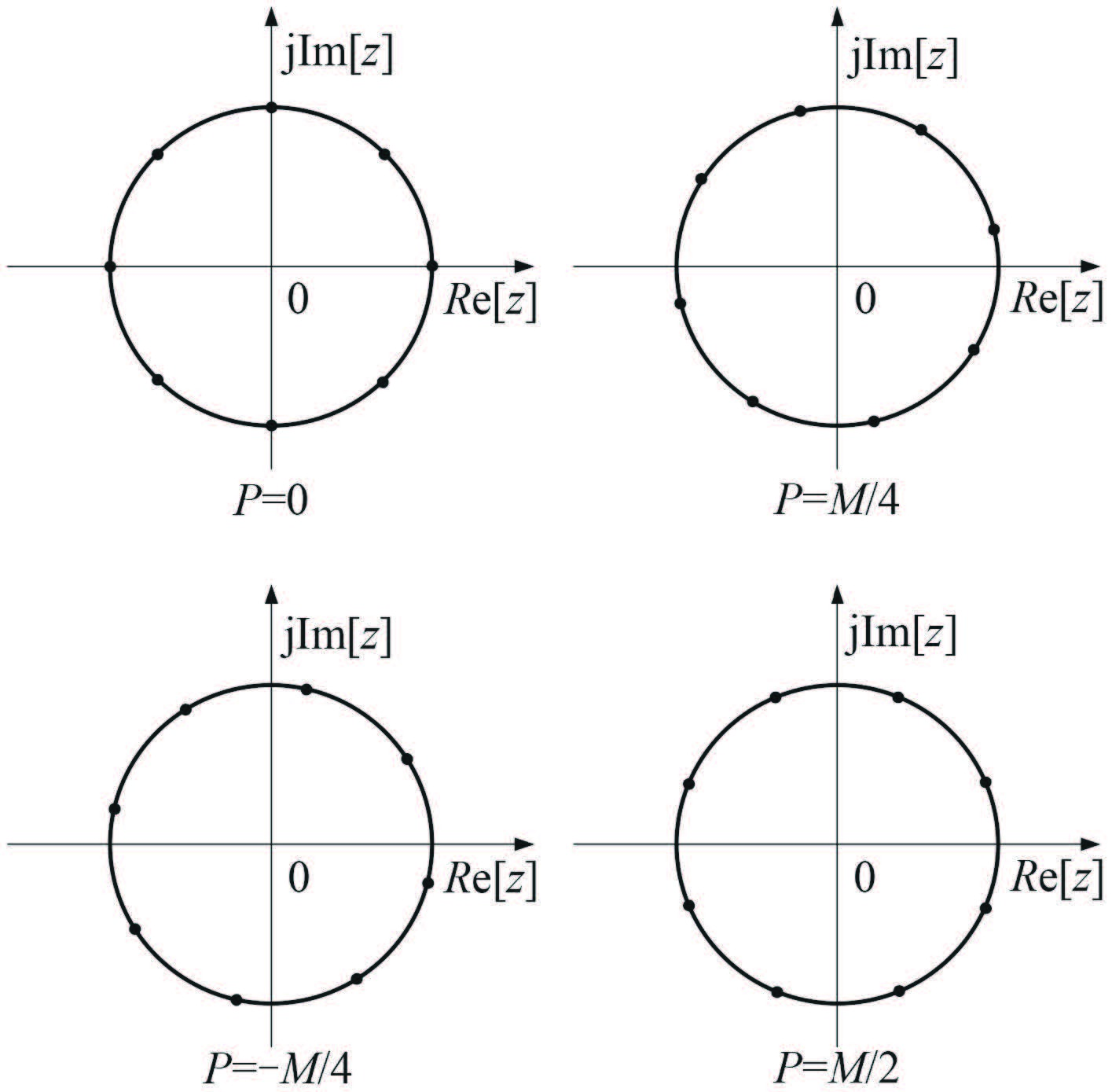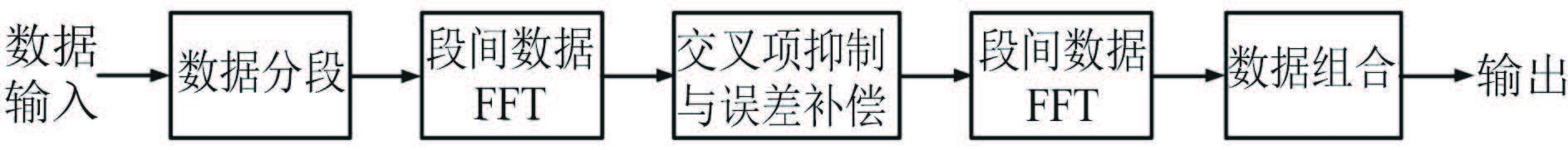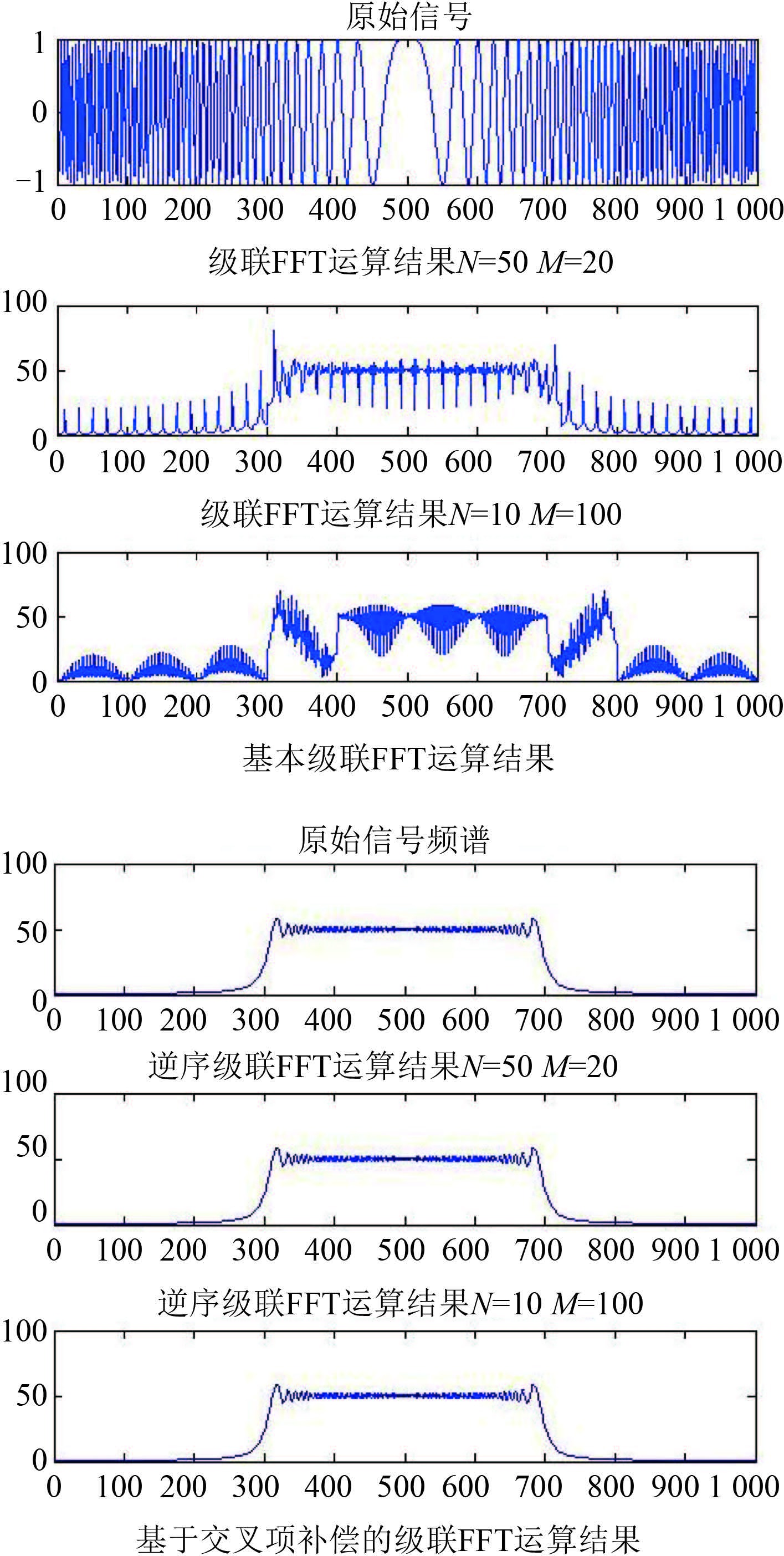﻿ 一种新的级联FFT算法
 舰船科学技术2016, Vol. 38Issue (5): 60-63PDF

A new inverse order cascade FFT algorithm
ZHANG Da-wei
China National Electronics Import and Export Corporation, Beijing 100037, China
Abstract: A new Inverse Order Cascade FFT (IOCFFT) algorithm is provided in this paper based on the analysis of the principle and shortage to the traditional Cascade FFT algorithm. The order of the traditional Cascade FFT algorithm is changed to reduce the processing load and energy leakage phenomenon. The inverse order of the traditional Cascade FFT is adopted in this new algorithm and intersection factor compensation is done between the two FFT stages. The phenomenon of grating lobes and high processing load is avoided in this new method. The validity and feasibility of the new algorithm is tested by deduction of the formulation and simulation of the theory.
Key words: cascade FFT     inverse order cascade FFT     intersection factor
0 引言

1 基本级联 FFT 的算法原理

M × N 点序列信号 xn + mN），n = 0,1,2,…,N-1；m = 0,1,2,…,M-1，利用级联 FFT 进行处理可以表示为 2 级 FFT 串联的变现形式。通过矩阵化，一维序列可以表示为二维 M × N 矩阵的形式，对于 N 点表示的数据进行 FFT 运算可以表示为：

 ${{X}_{m}}(q)=\sum\limits_{n=0}^{N-1}{x(n+mN){{e}^{-j2\pi nq/N}}}=\text{FF}{{\text{T}}_{n}}[x(n+mN)].$ (1)

 \begin{align} & X'(p+qM)=\text{FF}{{\text{T}}_{m}}\{\text{FF}{{\text{T}}_{n}}[x(n+mN)]\}= \\ & \sum\limits_{m=0}^{M-1}{{{e}^{-j2\pi mp/M}}\left( \sum\limits_{n=0}^{N-1}{x(n+mN){{e}^{-j2\pi nq/N}}} \right)}, \\ \end{align} (2)

 $X(p+qM)=\sum\limits_{n=0}^{N-1}{\sum\limits_{m=0}^{M-1}{x(n+mN){{e}^{-j2\pi \left( p+qM \right)\left( n+mN \right)/MN}}}},$ (3)

 $\begin{array}{*{35}{l}} {{X}_{1}}(p+qM)=\sum\limits_{m=0}^{M-1}{{{e}^{-j2\pi mp/M}}\left( \sum\limits_{n=0}^{N-1}{x(n+mN){{e}^{-j2\pi nq/N}}} \right)}= \\ \text{FF}{{\text{T}}_{m}}\{\text{FF}{{\text{T}}_{n}}[x(n+mN)]\}.\quad \quad \quad \quad \quad \\ \end{array}$ (4)

2 基于交叉项补偿的级联 FFT 算法 2.1 级联 FFT 算法的局限性

 ${{e}^{-j2\pi n\left( q+p/M \right)/N}}.$ (5)

2.2 基于交叉项补偿的级联 FFT 算法

 $\begin{array}{*{35}{l}} {{X}_{1}}(p+qM)=\sum\limits_{n=0}^{N-1}{{{e}^{-j2\pi nq/N}}\sum\limits_{m=0}^{M-1}{x(n+mN){{e}^{-j2\pi mp/M}}}}= \\ \text{FF}{{\text{T}}_{n}}\{\text{FF}{{\text{T}}_{m}}[x(n+mN)]\}. \\ \end{array}$ (6)

 \begin{align} & X(p+qM)=\sum\limits_{n=0}^{N-1}{\sum\limits_{m=0}^{M-1}{x(n+mN){{e}^{-j2\pi n\left( q+p/M \right)/N}}}}\times {{e}^{-j2\pi mp/M}}= \\ & \sum\limits_{n=0}^{N-1}{{{e}^{-j2\pi n\left( q+p/M \right)/N}}\sum\limits_{m=0}^{M-1}{x(n+mN){{e}^{-j2\pi mp/M}}}}= \\ & \sum\limits_{n=0}^{N-1}{{{e}^{-j2\pi nq/N}}{{e}^{-j2\pi np/MN}}X(p,n)}. \\ \end{align} (7)图 1 基于交叉项补偿的 FFT 算法示意图 Fig. 1 Diagram of FFT algorithm based on intersection factor compensation图 2 交叉项影响相角示意图 Fig. 2 Diagram of influence of intersection factor to the phase angle

3 算法仿真及性能分析图 3 基于交叉项补偿的级联 FFT 处理流程图 Fig. 3 Flow chart of Cascade FFT algorithm based on intersection factor compensation图 4 新算法进行处理后的频谱分析对比 Fig. 4 Spectrum comparison of new algorithm表 1 两种算法的运算量比较 Tab.1 Calculation amount comparison of two algorithm

4 结语

  PERRY R P, KAISER H W. Digital step transform approach to airborne radar processing[C]//Proceedings of IEEE National Aerospace and Electronics Conference. New York:IEEE, 1973:280-287.  YIP P C Y. Some aspects of the Zoom transform[J]. IEEE Transactions on Computers , 1976, C-25 (3) :287–296. DOI:10.1109/TC.1976.5009255  FJELL P O, LUNDE E B. A modified cascade fast Fourier transform in a spectrum analysing system[C]//Proceedings of IEEE international conference on ICASSP '77 acoustics, speech, and signal processing. Hartford, CT, USA:IEEE, 1997, (2):873-876.  HOYER E A, STORK R F. The Zoom FFT using complex modulation[C]//Proceedings of IEEE international conference on ICASSP '77 acoustics, speech, and signal processing. Hartford, CT, USA:IEEE, 1977, (2):78-81.  WU K H, VANT M R. Extensions to the step transform SAR processing technique[J]. IEEE Transactions on Aerospace and Electronic Systems , 1985, AES-21 (3) :338–344. DOI:10.1109/TAES.1985.310563  MCGOEY-SMITH A D, VANT M R. Modification of the SAR Step Transform algorithm[J]. IEEE Transactions on Aerospace and Electronic Systems , 1992, 28 (3) :666–674. DOI:10.1109/7.256289  MOREIRA A. Real-time Synthetic aperture radar (SAR) processing with a new subaperture approach[J]. IEEE Transactions on Geoscience and Remote Sensing , 1992, 30 (4) :714–722. DOI:10.1109/36.158865  SUN X B, YEO T S, ZHANG C B, et al. Time-varying step-transform algorithm for high squint SAR imaging[J]. IEEE Transactions on Geoscience and Remote Sensing , 1999, 37 (6) :2668–2677. DOI:10.1109/36.803414  YEO T S, TAN N L, ZHANG C B, et al. A new subaperture approach to high squint SAR processing[J]. IEEE Transactions on Geoscience and Remote Sensing , 2001, 39 (5) :954–968. DOI:10.1109/36.921413